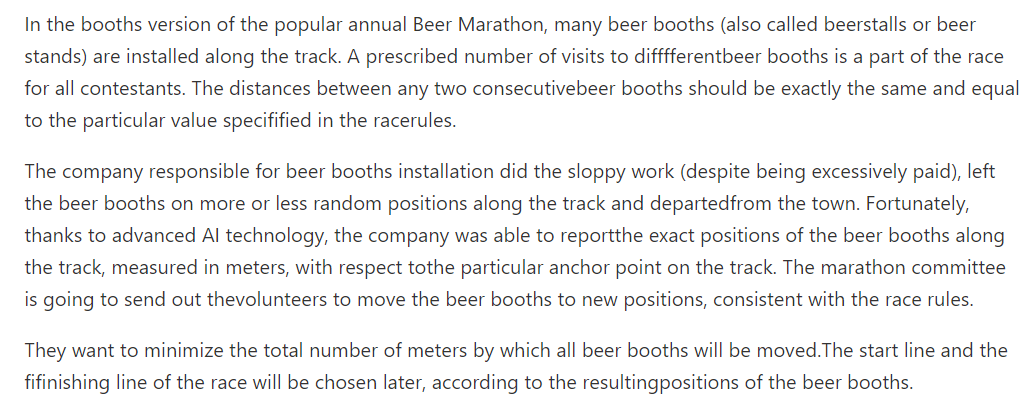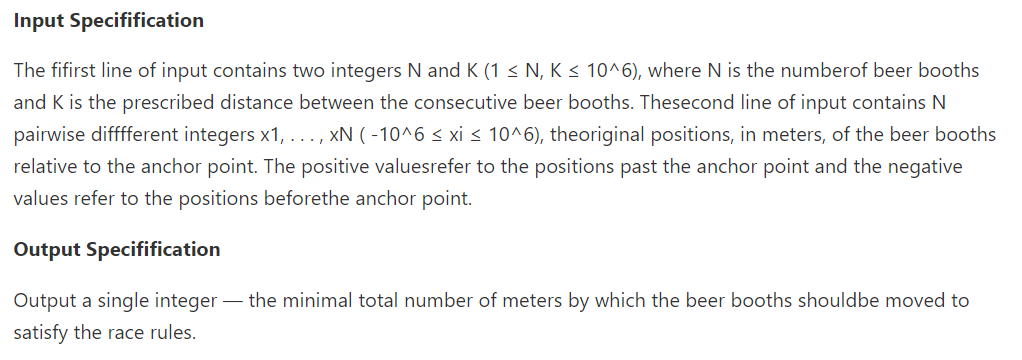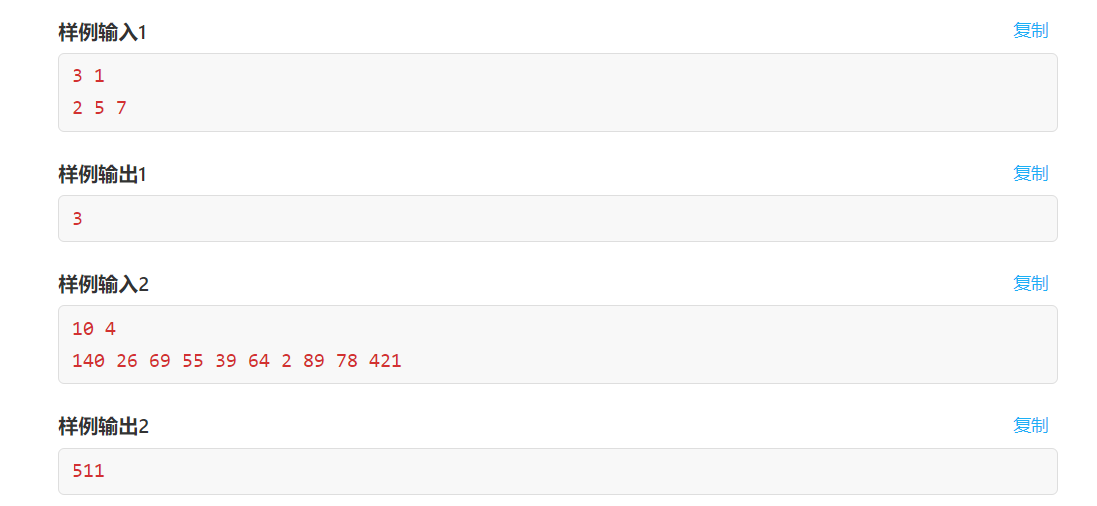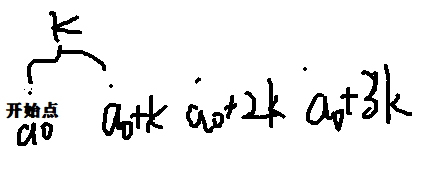## F. Beer Marathon（二分+迭代+贪心）AC代码：

``````#include<bits/stdc++.h>
using namespace std;
typedef long long ll;
ll n,k;
ll a;
ll sum(ll d){

//这个函数就是用来计算初始值和最终值的差值的和，也就是最终移动的距离
ll res=0;
for(int i=0;i<n;i++){

res+=abs(a[i]-d);
d+=k;
}
return res;
}
int main(){

cin>>n>>k;
for(ll i=0;i<n;i++)cin>>a[i];
sort(a,a+n);//题目输入并没有顺序，所以需要排序
ll l=-1e12,r=1e12;//二分起点坐标
ll t1,t2;
ll ans=1e18;//记录答案
for(ll i=0;i<100;i++){

ll L=l+(r-l)/3,R=r-(r-l)/3;//这里为什么不用L=l,R=r？就是迭代的知识，只不过这点确实不好想
t1=sum(L);
t2=sum(R);
if(t1<t2){

r=R;
}else {

l=L;
}
ans=min(ans,min(t1,t2));
//cout<<t1<<t2<<endl;
}
cout<<ans<<endl;
return 0;
}

``````